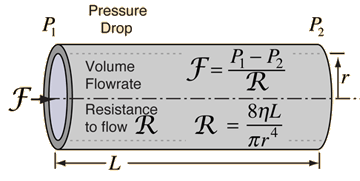How is flow rate related to viscosity?

May 24, 2017

Flow rate is inversely proportional to viscosity.

Explanation:

The relation is called Poiseuille's law, which describes the smooth flow of a fluid along a tube.The flow rate $F$ is proportional to the pressure drop Δp = p_1 – p_2 divided by $R$, the resistance to flow.

color(blue)(bar(ul(|color(white)(a/a) F = (Δp)/R = (p_1 – p_2)/R color(white)(a/a)|)))

The resistance to flow in turn is directly proportional to the viscosity $\eta$.

color(blue)(bar(ul(|color(white)(a/a) R = (8etaL)/(πr^4)color(white)(a/a)|)))" "

Combining the two formulas gives Poiseuille's law:

color(blue)(bar(ul(|color(white)(a/a)F = (πr^4Δp)/(8etaL)color(white)(a/a)|)))" "

If r, Δp, and $L$ are constant, we can write

$F = \frac{k}{\eta}$

Thus, the flow rate is inversely proportional to the viscosity.